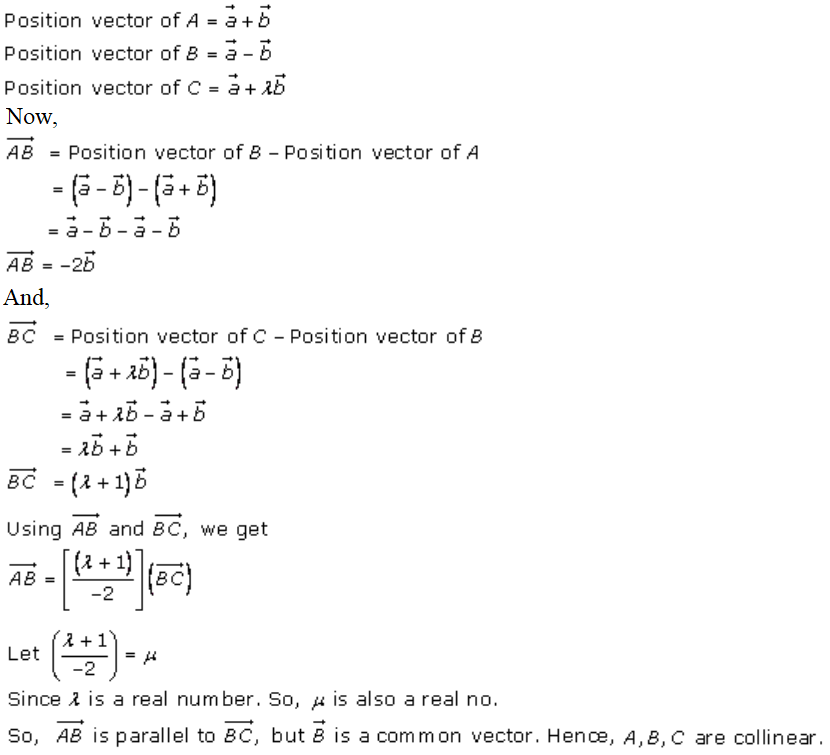# RD Sharma Solutions Class 12 Algebra Of Vectors Exercise 23.7

RD Sharma Solutions for Class 12 Maths Chapter 23 Algebra of Vectors Exercise 23.7 are provided here for students to kick-start their board exam preparations. Experts at BYJU’S have designed these solutions in a simple language to meet the needs of all types of students. The RD Sharma Solutions for this particular exercise is readily accessible in PDF format, which can be easily downloaded by the students, from the link given below.

## Download PDF of Rd Sharma Solution for Class 12 Maths Chapter 23 Exercise 7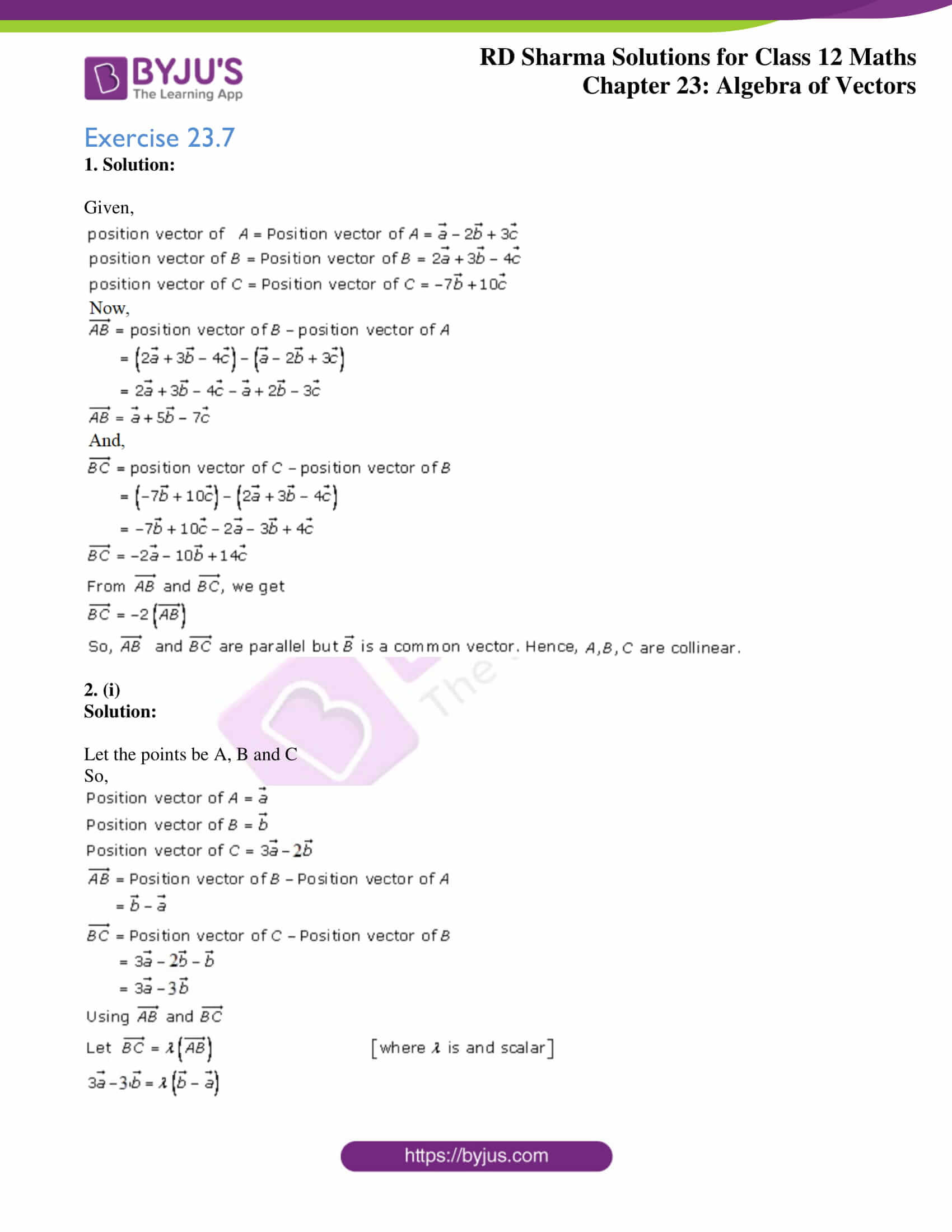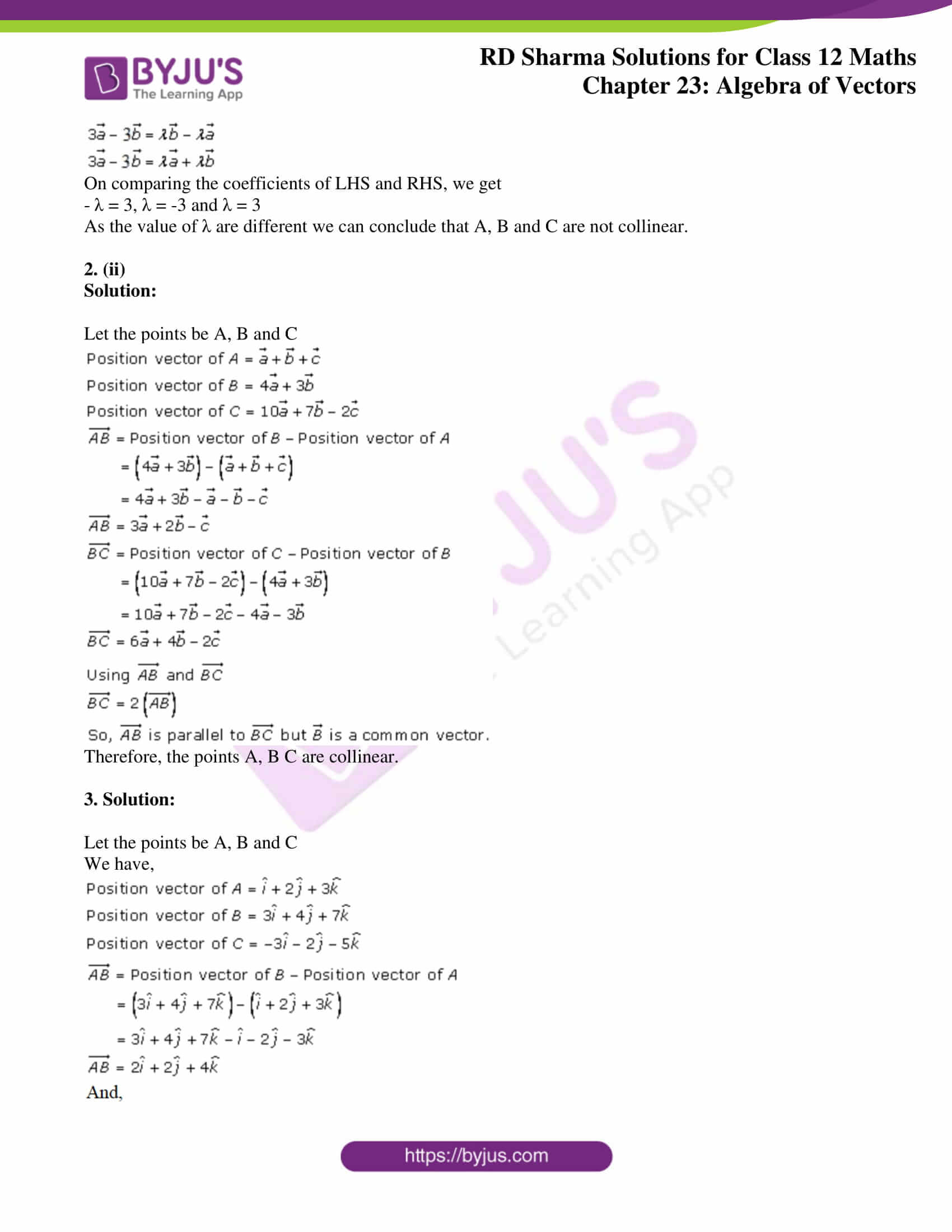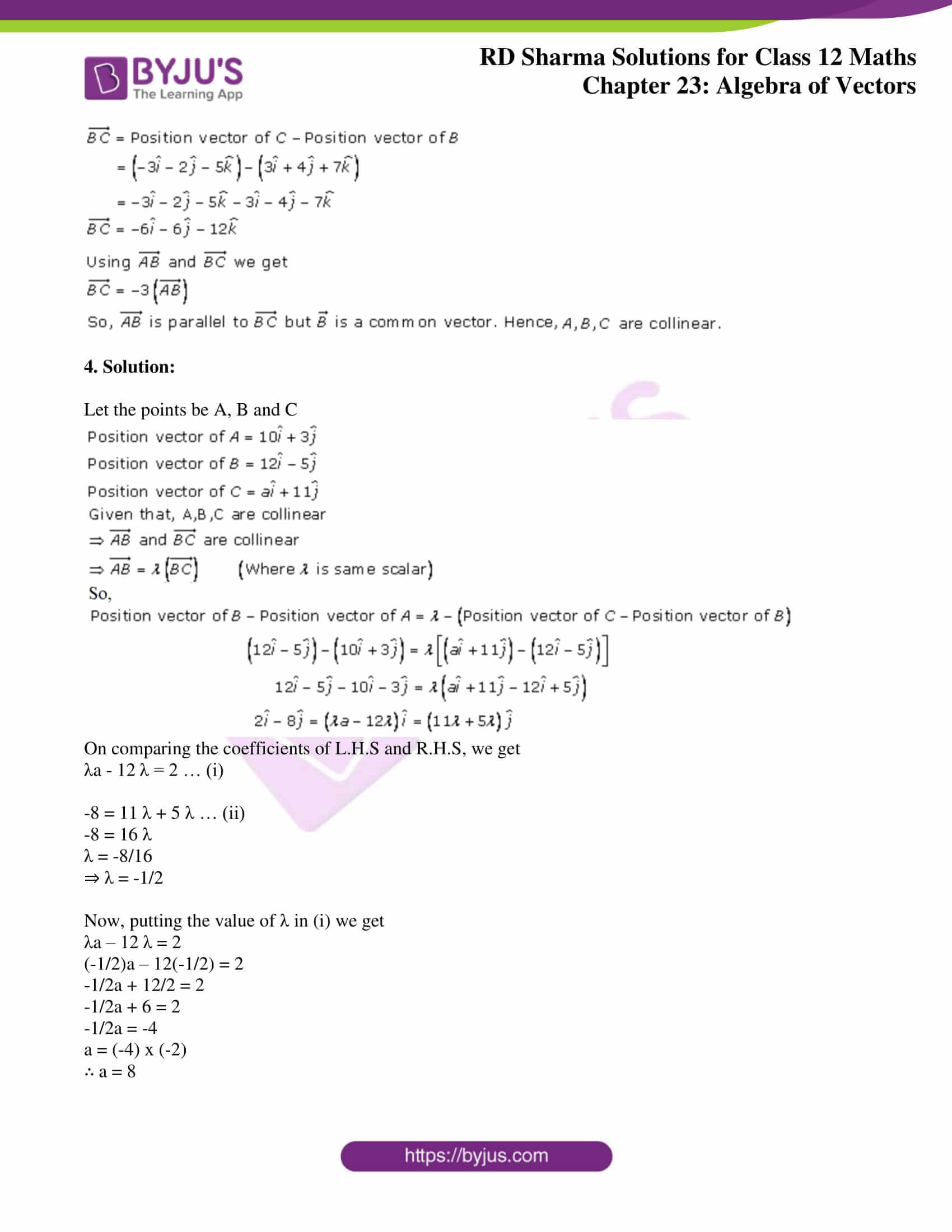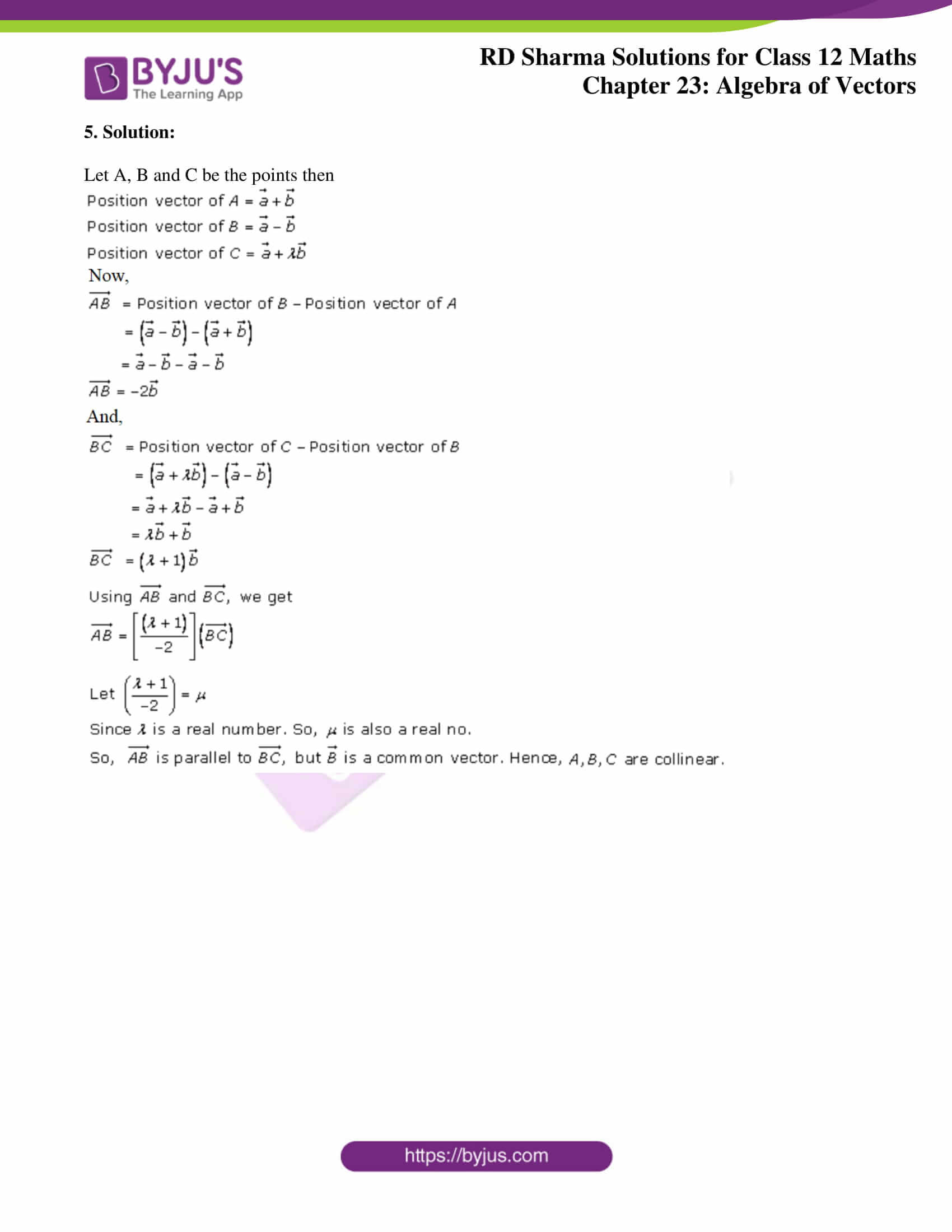### Access Answers for Rd Sharma Solution Class 12 Maths Chapter 23 Exercise 7

Exercise 23.7

1. Solution:

Given,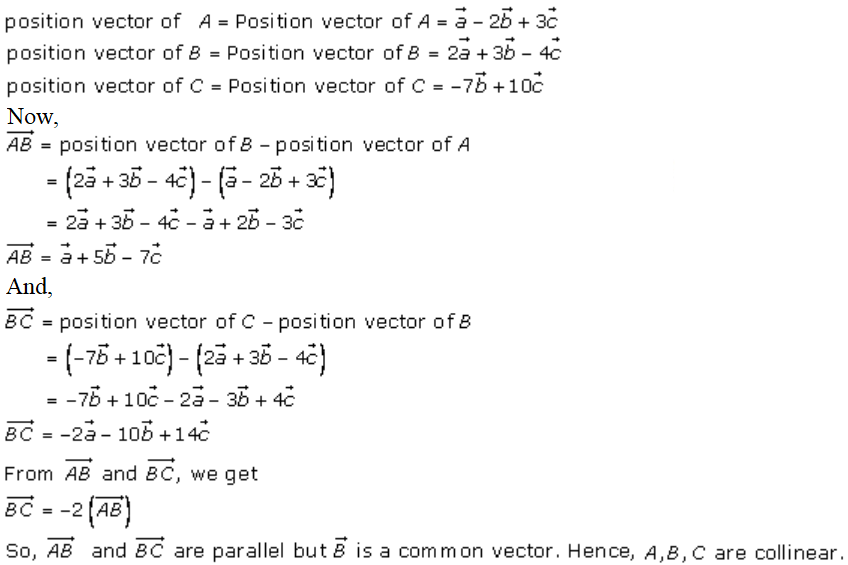2. (i)

Solution:

Let the points be A, B and C

So,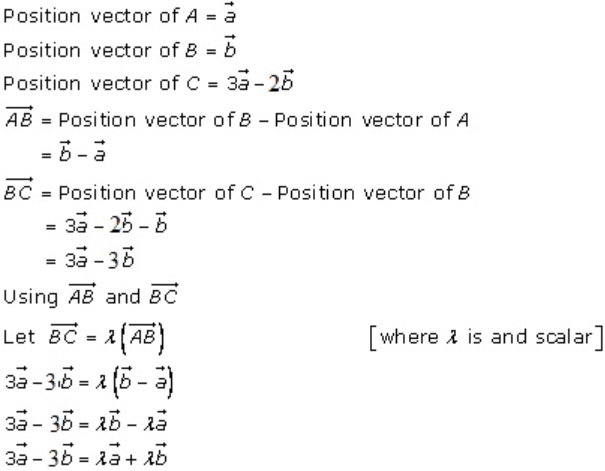On comparing the coefficients of LHS and RHS, we get

– λ = 3, λ = -3 and λ = 3

As the value of λ are different we can conclude that A, B and C are not collinear.

2. (ii)

Solution:

Let the points be A, B and C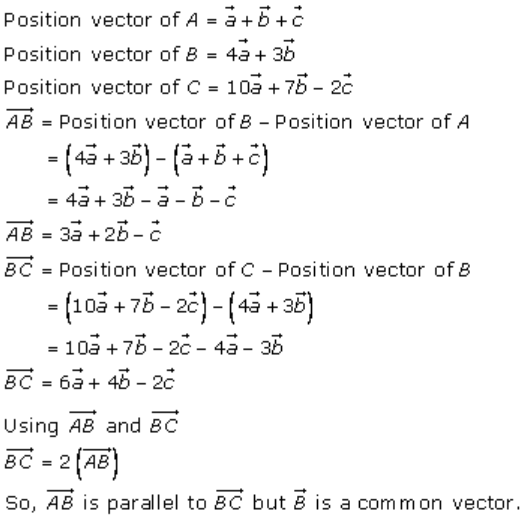Therefore, the points A, B C are collinear.

3. Solution:

Let the points be A, B and C

We have,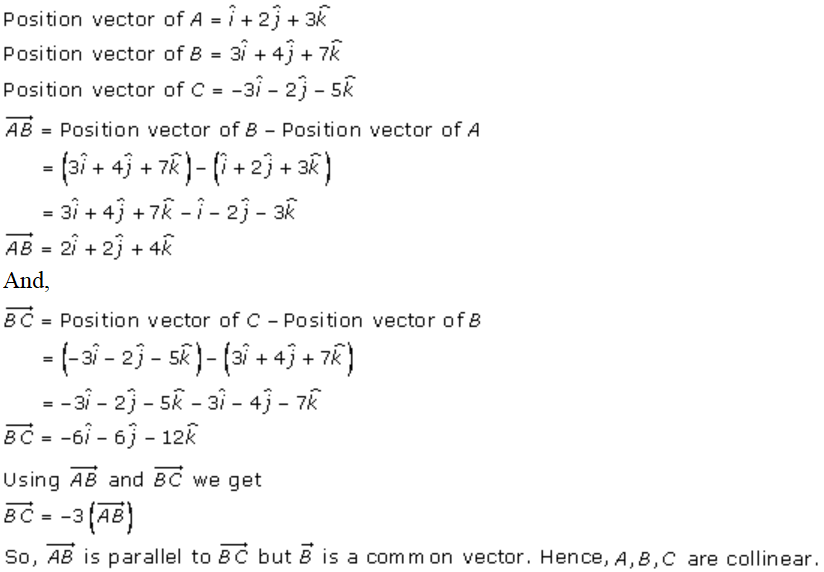4. Solution:

Let the points be A, B and C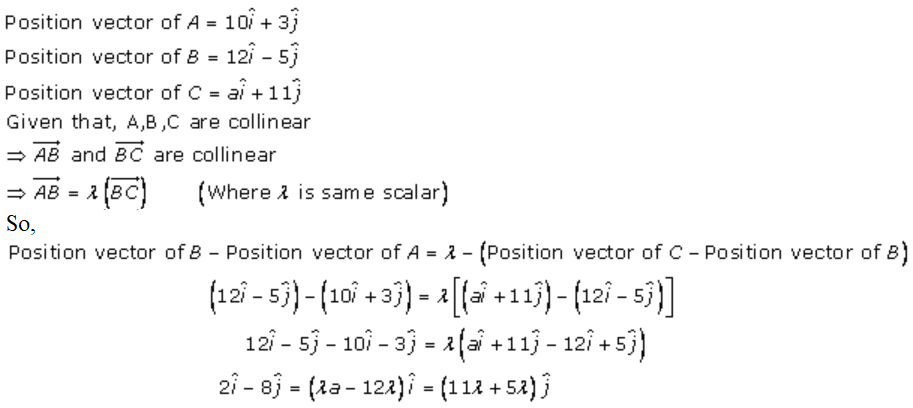On comparing the coefficients of L.H.S and R.H.S, we get

λa – 12 λ = 2 … (i)

-8 = 11 λ + 5 λ … (ii)

-8 = 16 λ

λ = -8/16

⇒ λ = -1/2

Now, putting the value of λ in (i) we get

λa – 12 λ = 2

(-1/2)a – 12(-1/2) = 2

-1/2a + 12/2 = 2

-1/2a + 6 = 2

-1/2a = -4

a = (-4) x (-2)

∴ a = 8

5. Solution:

Let A, B and C be the points then